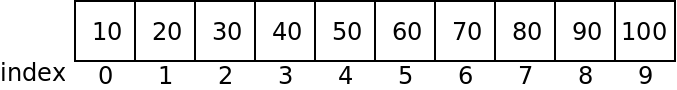# Arrays

## Introduction

So far we have looked at programs that use scalar variables: ones that can only store one value at a time. There is a lot we can do with these variables, but some things require arrays.

An array is a variable that can hold multiple different values at the same time. They can be used for storing larger sets of information such as:

• The phone numbers of multiple friends.
• The set of prime numbers less than 100.
• The prices of multiple items for sale.

## Creating Arrays in Java

To declare an array in Java, we use the following syntax:


<type> <name>[] = new <type>[<size>];

For example, to declare an array of 100 strings called "names":

String names = new String;


## Indexing Arrays

An array is accessed by indexing it. This is done with placing the desired index withing square brackets after the array name:


public class Arrays {

public static void main(String args[]) {
String names[] = new String;

names = "Allen";
names = "Bob";
names = "Claire";
names = "Donald";
names = "Ellen";

System.out.println("The second person is " + names);
}
}


## Example: Simple Statistics

Given the following code:


import java.util.Random;

class Stats {

// some constants "final" means cannot be changed
public static final int SIZE = 25;
public static final int MAX = 100;

public static void main(String args[]) {
// create the array
int array[] = new int[SIZE];

// fill it up with random numbers
Random generator = new Random();
for (int i = 0; i < SIZE; i++) {
array[i] = generator.nextInt(MAX);
}

}
}


How could we find the smallest, largest, and average value in the array?





## Initializing Arrays

If we know ahead of time what values we want in an array, we can initialize it directly using the following syntax:


int numbers[] = new int[] {10, 20, 30, 40, 50, 60, 70, 80, 90, 100};


Note that the size of the array is not specified here. The compiler will figure out that the array needs 10 elements.

## Array Storage

Notice that array indices start at zero and go through the size - 1. A common mistake is an "off by one" error in which we mistake the bounds of an array.

Java stores arrays contiguously in memory. The following array:


int numbers [] = {10, 20, 30, 40, 50, 60, 70, 80, 90, 100};


Will be stored as follows:If we access too far to the left, or too far to the right of an array, what happens?


class Fail {
public static void main(String args[]) {
// create the array
int array[] = new int[] {1, 2, 3};

System.out.println("Iterm 3 is: " + array);
}
}


You can find an arrays length for any array with the ".length" member:


class Length {

public static void main(String args[]) {
// create the array
int array[] = new int[] {1, 2, 3};

System.out.println("The length of array is: " + array.length);
}
}


Note that the length minus one is the last element of an array!

## Passing Arrays to Functions

The following example passes an array to a function:


class Passing {
public static void printArray(int data[]) {
System.out.print("[");
for (int i= 0; i < data.length; i++) {
System.out.print(data[i] + ", ");
}
System.out.println("\b\b]");
}

public static void main(String args[]) {
// create the array
int array[] = new int[] {1, 2, 3};
printArray(array);
}
}


When passing an array, the size is not included in the parameter. Just empty brackets.

Functions may also return arrays:


import java.util.Random;

class Return {

public static void printArray(int data[]) {
System.out.print("[");
for (int i= 0; i < data.length; i++) {
System.out.print(data[i] + ", ");
}
System.out.println("\b\b]");
}

public static int[] getRandomData(int size, int max) {
int data[] = new int[size];
Random generator = new Random();
for (int i = 0; i < size; i++) {
data[i] = generator.nextInt(max);
}
return data;
}

public static void main(String args[]) {
printArray(getRandomData(10, 10));
}
}


When passing an array to a function, the address of the array in memory is passed. This means changes to the array take effect on the array that is passed in:


import java.util.Random;

class Effects {

public static void printArray(int data[]) {
System.out.print("[");
for (int i= 0; i < data.length; i++) {
System.out.print(data[i] + ", ");
}
System.out.println("\b\b]");
}

public static void clearArray(int data[]) {
for (int i= 0; i < data.length; i++) {
data[i] = 0;
}
}

public static int[] getRandomData(int size, int max) {
int data[] = new int[size];
Random generator = new Random();
for (int i = 0; i < size; i++) {
data[i] = generator.nextInt(max);
}
return data;
}

public static void main(String args[]) {
int array[] = getRandomData(10, 10);
printArray(array);
clearArray(array);
printArray(array);
}
}


## Strings as Arrays

String variables in Java can actually be accessed somewhat like arrays. Instead of brackets, we can use the ".charAt" function:


class Strings {

public static void main(String args[]) {
String name = "Ian Finlayson";
for (int i = 0; i < name.length(); i++) {
System.out.println(name.charAt(i));
}
}
}


Note that the characters in a String cannot be changed, however!